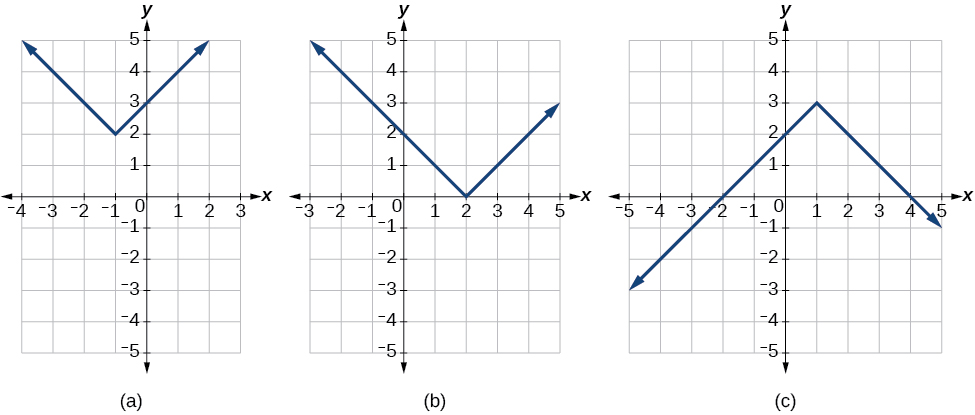# 3.6 Absolute value functions  (Page 2/3)

 Page 2 / 3

If we couldn’t observe the stretch of the function from the graphs, could we algebraically determine it?

Yes. If we are unable to determine the stretch based on the width of the graph, we can solve for the stretch factor by putting in a known pair of values for $\text{\hspace{0.17em}}x\text{\hspace{0.17em}}$ and $\text{\hspace{0.17em}}f\left(x\right).$

$f\left(x\right)=a|x-3|-2$

Now substituting in the point (1, 2)

$\begin{array}{ccc}\hfill 2& =& a|1-3|-2\hfill \\ \hfill 4& =& 2a\hfill \\ \hfill a& =& 2\hfill \end{array}$

Write the equation for the absolute value function that is horizontally shifted left 2 units, is vertically flipped, and vertically shifted up 3 units.

$f\left(x\right)=-|x+2|+3$

Do the graphs of absolute value functions always intersect the vertical axis? The horizontal axis?

Yes, they always intersect the vertical axis. The graph of an absolute value function will intersect the vertical axis when the input is zero.

No, they do not always intersect the horizontal axis. The graph may or may not intersect the horizontal axis, depending on how the graph has been shifted and reflected. It is possible for the absolute value function to intersect the horizontal axis at zero, one, or two points (see [link] ).(a) The absolute value function does not intersect the horizontal axis. (b) The absolute value function intersects the horizontal axis at one point. (c) The absolute value function intersects the horizontal axis at two points.

## Solving an absolute value equation

In Other Type of Equations , we touched on the concepts of absolute value equations. Now that we understand a little more about their graphs, we can take another look at these types of equations. Now that we can graph an absolute value function, we will learn how to solve an absolute value equation. To solve an equation such as $\text{\hspace{0.17em}}8=|2x-6|,\text{\hspace{0.17em}}$ we notice that the absolute value will be equal to 8 if the quantity inside the absolute value is 8 or -8. This leads to two different equations we can solve independently.

$\begin{array}{ccccccc}\hfill 2x-6& =& 8\hfill & \phantom{\rule{1em}{0ex}}\text{or}\phantom{\rule{1em}{0ex}}& \hfill 2x-6& =& -8\hfill \\ \hfill 2x& =& 14\hfill & & \hfill 2x& =& -2\hfill \\ \hfill x& =& 7\hfill & & \hfill x& =& -1\hfill \end{array}$

Knowing how to solve problems involving absolute value functions is useful. For example, we may need to identify numbers or points on a line that are at a specified distance from a given reference point.

An absolute value equation is an equation in which the unknown variable appears in absolute value bars. For example,

$\begin{array}{l}|x|=4,\hfill \\ |2x-1|=3,\text{or}\hfill \\ |5x+2|-4=9\hfill \end{array}$

## Solutions to absolute value equations

For real numbers $A$ and $B$ , an equation of the form $|A|=B,$ with $B\ge 0,$ will have solutions when $A=B$ or $A=-B.$ If $B<0,$ the equation $|A|=B$ has no solution.

Given the formula for an absolute value function, find the horizontal intercepts of its graph .

1. Isolate the absolute value term.
2. Use $\text{\hspace{0.17em}}|A|=B\text{\hspace{0.17em}}$ to write $\text{\hspace{0.17em}}A=B\text{\hspace{0.17em}}$ or $\text{\hspace{0.17em}}\mathrm{-A}=B,\text{\hspace{0.17em}}$ assuming $\text{\hspace{0.17em}}B>0.$
3. Solve for $\text{\hspace{0.17em}}x.\text{\hspace{0.17em}}$

## Finding the zeros of an absolute value function

For the function $\text{\hspace{0.17em}}f\left(x\right)=|4x+1|-7,$ find the values of $\text{\hspace{0.17em}}x\text{\hspace{0.17em}}$ such that $\text{\hspace{0.17em}}f\left(x\right)=0.$

The function outputs 0 when $\text{\hspace{0.17em}}x=\frac{3}{2}\text{\hspace{0.17em}}$ or $\text{\hspace{0.17em}}x=-2.$ See [link] .

For the function $\text{\hspace{0.17em}}f\left(x\right)=|2x-1|-3,$ find the values of $\text{\hspace{0.17em}}x\text{\hspace{0.17em}}$ such that $\text{\hspace{0.17em}}f\left(x\right)=0.$

$x=-1\text{\hspace{0.17em}}$ or $\text{\hspace{0.17em}}\text{\hspace{0.17em}}x=2$

Should we always expect two answers when solving $\text{\hspace{0.17em}}|A|=B?$

No. We may find one, two, or even no answers. For example, there is no solution to $\text{\hspace{0.17em}}2+|3x-5|=1.$

#### Questions & Answers

A laser rangefinder is locked on a comet approaching Earth. The distance g(x), in kilometers, of the comet after x days, for x in the interval 0 to 30 days, is given by g(x)=250,000csc(π30x). Graph g(x) on the interval [0, 35]. Evaluate g(5)  and interpret the information. What is the minimum distance between the comet and Earth? When does this occur? To which constant in the equation does this correspond? Find and discuss the meaning of any vertical asymptotes.
Kaitlyn Reply
The sequence is {1,-1,1-1.....} has
amit Reply
circular region of radious
Kainat Reply
how can we solve this problem
Joel Reply
Sin(A+B) = sinBcosA+cosBsinA
Eseka Reply
Prove it
Eseka
Please prove it
Eseka
hi
Joel
June needs 45 gallons of punch. 2 different coolers. Bigger cooler is 5 times as large as smaller cooler. How many gallons in each cooler?
Arleathia Reply
7.5 and 37.5
Nando
find the sum of 28th term of the AP 3+10+17+---------
Prince Reply
I think you should say "28 terms" instead of "28th term"
Vedant
the 28th term is 175
Nando
192
Kenneth
if sequence sn is a such that sn>0 for all n and lim sn=0than prove that lim (s1 s2............ sn) ke hole power n =n
SANDESH Reply
write down the polynomial function with root 1/3,2,-3 with solution
Gift Reply
if A and B are subspaces of V prove that (A+B)/B=A/(A-B)
Pream Reply
write down the value of each of the following in surd form a)cos(-65°) b)sin(-180°)c)tan(225°)d)tan(135°)
Oroke Reply
Prove that (sinA/1-cosA - 1-cosA/sinA) (cosA/1-sinA - 1-sinA/cosA) = 4
kiruba Reply
what is the answer to dividing negative index
Morosi Reply
In a triangle ABC prove that. (b+c)cosA+(c+a)cosB+(a+b)cisC=a+b+c.
Shivam Reply
give me the waec 2019 questions
Aaron Reply

### Read also:

#### Get the best Algebra and trigonometry course in your pocket!

Source:  OpenStax, Algebra and trigonometry. OpenStax CNX. Nov 14, 2016 Download for free at https://legacy.cnx.org/content/col11758/1.6
Google Play and the Google Play logo are trademarks of Google Inc.

Notification Switch

Would you like to follow the 'Algebra and trigonometry' conversation and receive update notifications?ByByBy Lakeima RobertsBy Anonymous UserBy RhodesBy Abishek DevarajBy Edward Biton